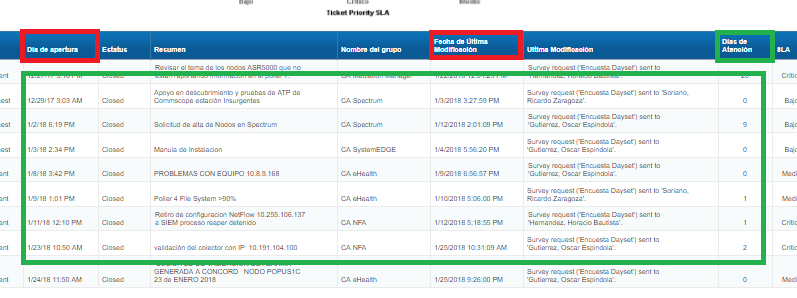CA Service Management

View Only

Dates RangeMarco Antonio Velazquez AldamaFeb 02, 2018 01:02 PMMarco Antonio Velazquez AldamaFeb 02, 2018 01:48 PM• 1.  Dates Range

Posted Feb 02, 2018 01:02 PM

hi everyone

Currently, I am working with a report in CABI 4.1, but my client needs to Add the days in which a ticket is open,

I added the following formula with Open Date and Resolve Date

=DaysBetween([Open Date];[Resolve Date])

but, the result is not right. some of the results show me as "0"Can you help me to complete my formula?
I will be very grateful

• 2.  Re: Dates Range

Posted Feb 02, 2018 01:48 PM

I tried with the following formulas, but the result its the same

=(Si(FormatoFecha([Resolve Date]; "dd-MM-yyyy") <> "") Then ((DíasEntre([Open Date];[Resolve Date])*24*60)+((EnNúmero(FormatoFecha([Resolve Date];"HH"))*60)-(EnNúmero(FormatoFecha([Open Date];"HH"))*60))+((EnNúmero(FormatoFecha([Resolve Date];"mm"))-(EnNúmero(FormatoFecha([Open Date];"mm")))))+((EnNúmero(FormatoFecha([Resolve Date];"ss"))/60)-((EnNúmero(FormatoFecha([Open Date];"ss")))/60)))/60 Else 0)/24

*************************************

=(If(FormatDate([Resolve Date]; "dd-MM-yyyy") <>"") Then Abs((((DaysBetween([Resolve Date];[Open Date])*1440)+((ToNumber(FormatDate([Resolve Date];"HH"))*60)-(ToNumber(FormatDate([Open Date];"HH"))*60))+((ToNumber(FormatDate([Resolve Date];"mm"))-(ToNumber(FormatDate([Openate];"mm")))))+((ToNumber(FormatDate([Resolve Date];"ss"))/60)-((ToNumber(FormatDate([Open Date];"ss"))/60))))/60)) Else -1)

• 3.  Re: Dates Range Best Answer

Posted Feb 05, 2018 11:11 AM

Hi Marco,

DaysBetween([Open Date]; [Resolve Date]) is the correct formula, however looking at your screenshot, the year and time format does not match between open date and resolve date.

Perhaps this is causing the issue?

Thanks,

Scott